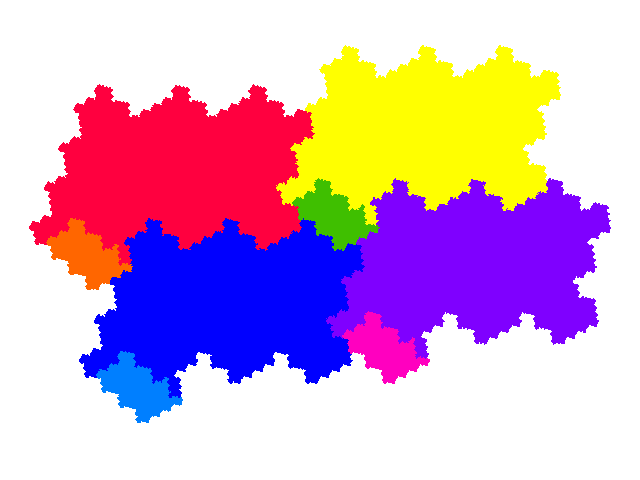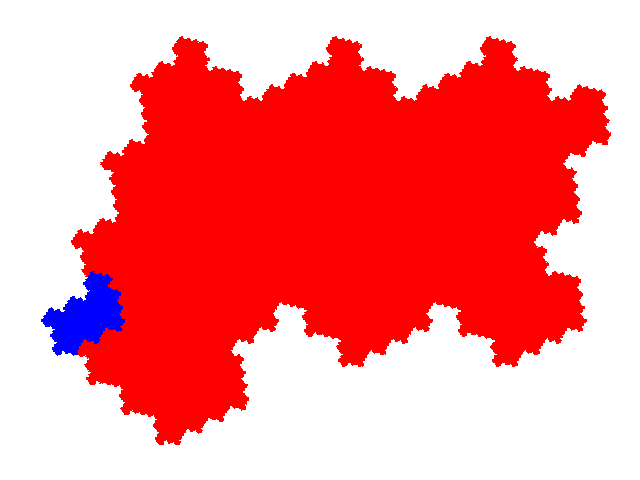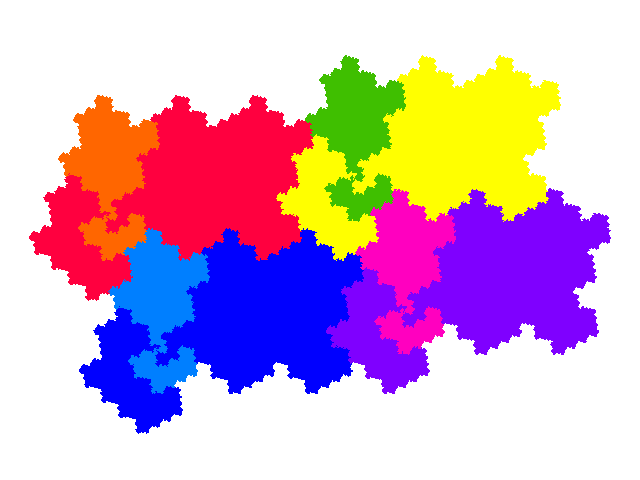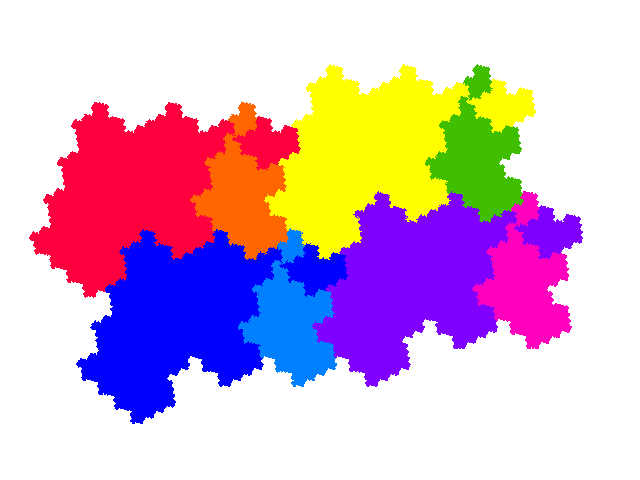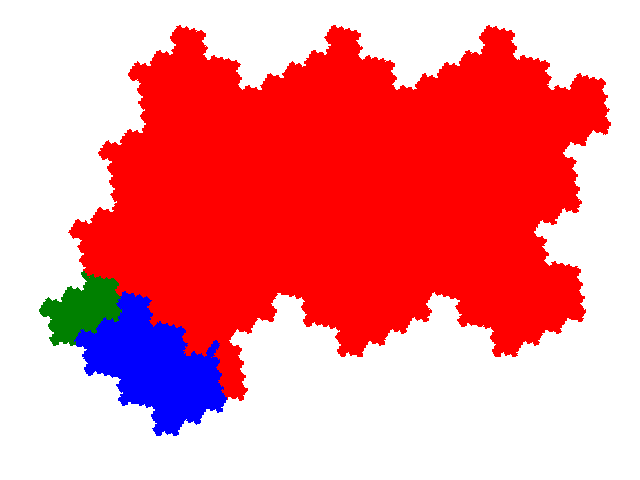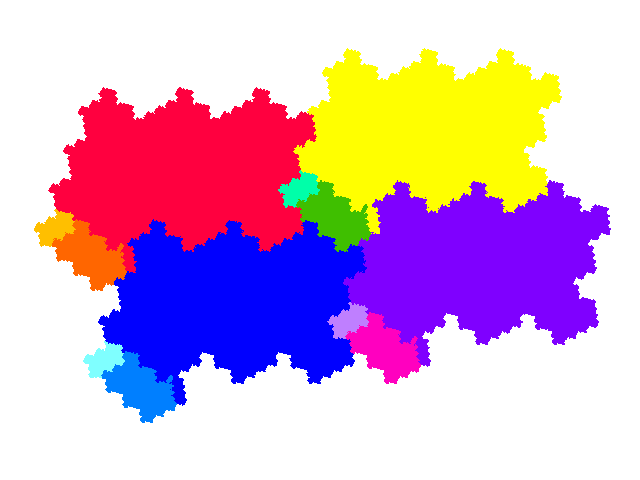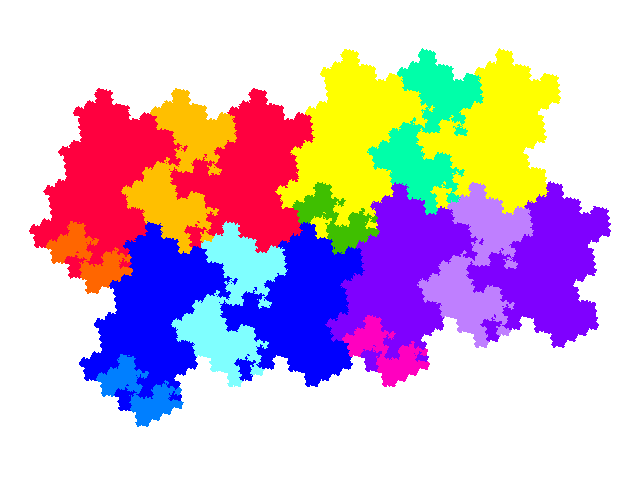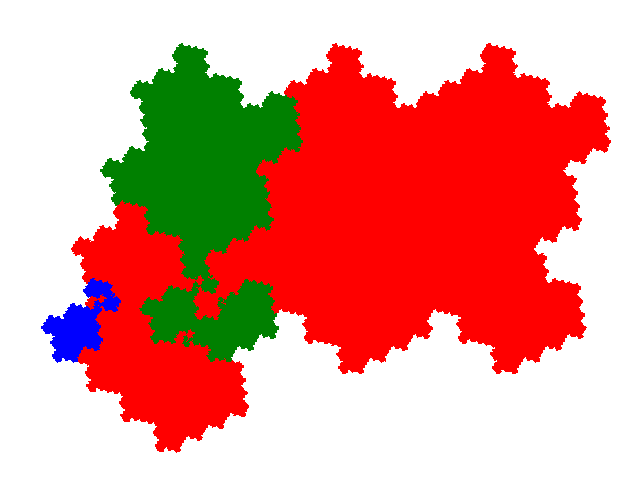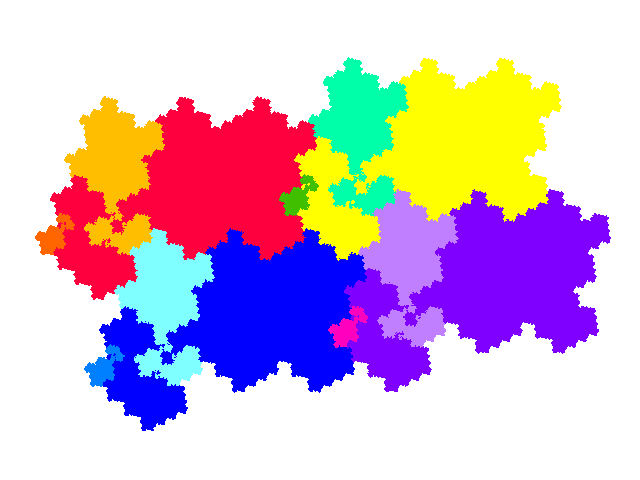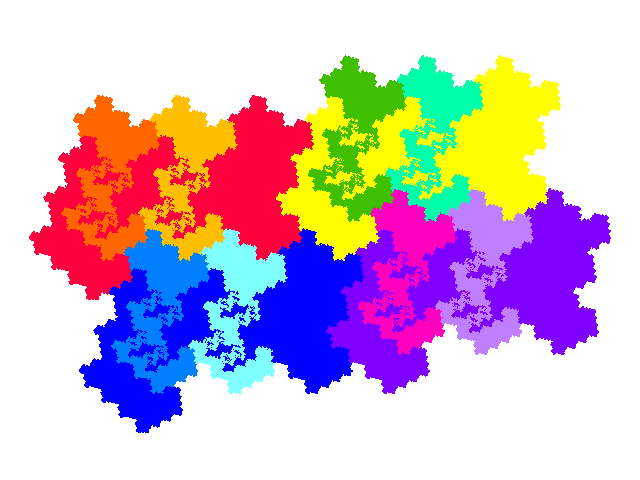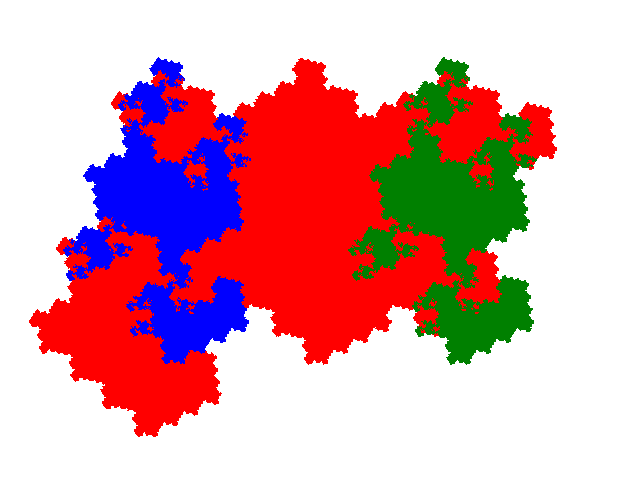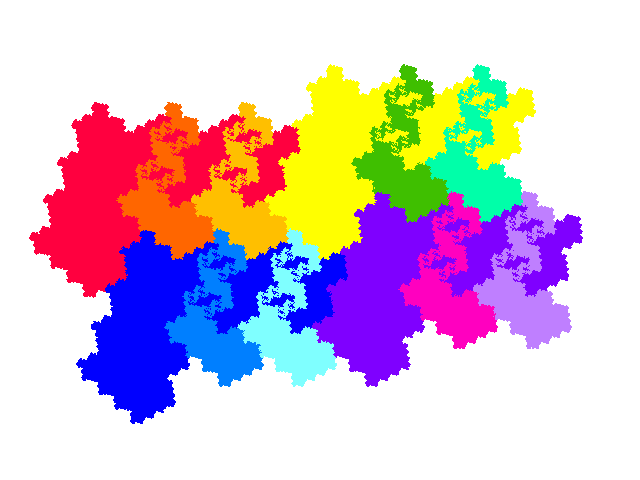# 12th Unit Cubic Pisot Tiles

A construction for tiles with dissection equation nx + x2 + x3 produces an order 5 12th unit cubic Pisot tile, with dissection equation 3x + x2 + x3.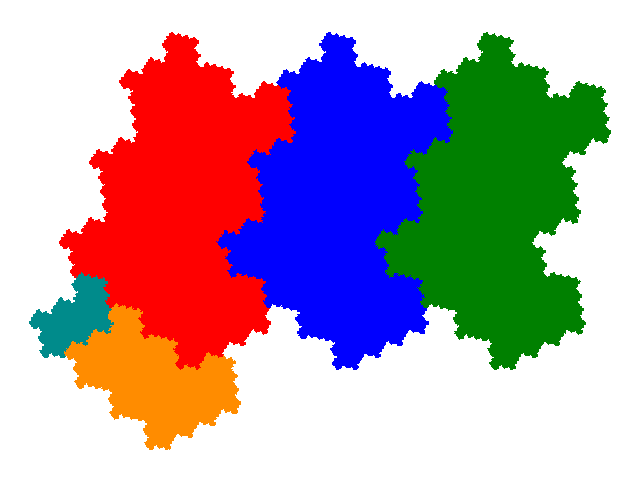From this 5 order 9 and 7 order 13 tiles can be derived. (The other 3 candidate order 13 tiles turn out to not be connected, though they still have a similarity dimension of 2 and tile the plane.) Order 17, 21, 25, 33, 37, 41, 45, 49, 61, 65, ... tiles can also be generated.

The order 9 tiles have dissection equations 3x + 4x3 + x5 + x6, 3x + x2 + 3x4 + x5 + x6 and 2x + 4x2 + 2x3 + x4 (thrice).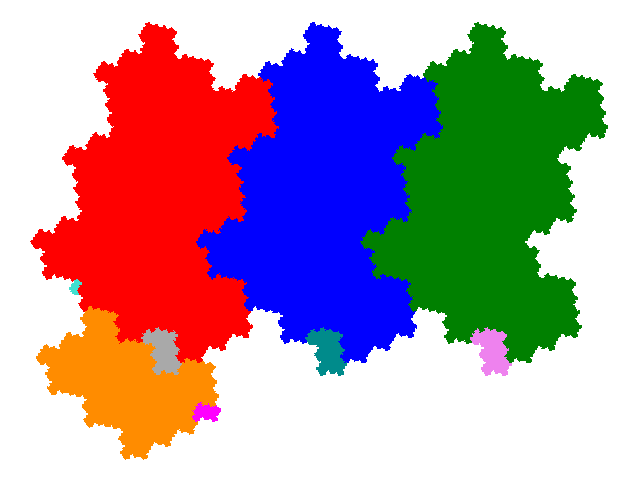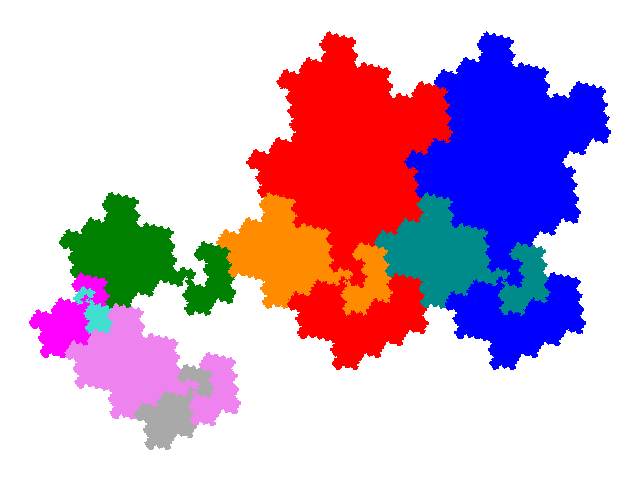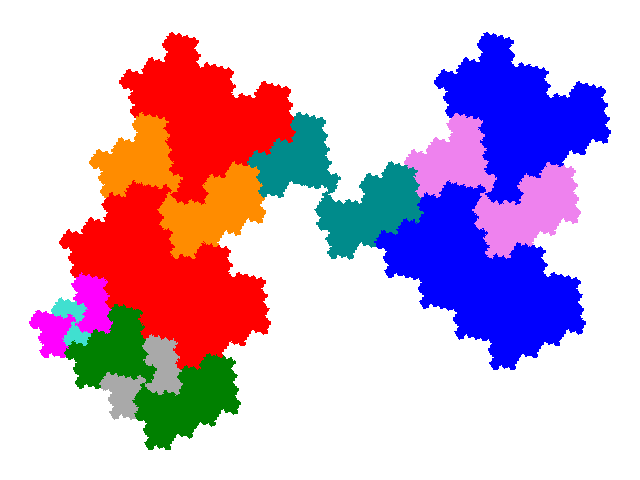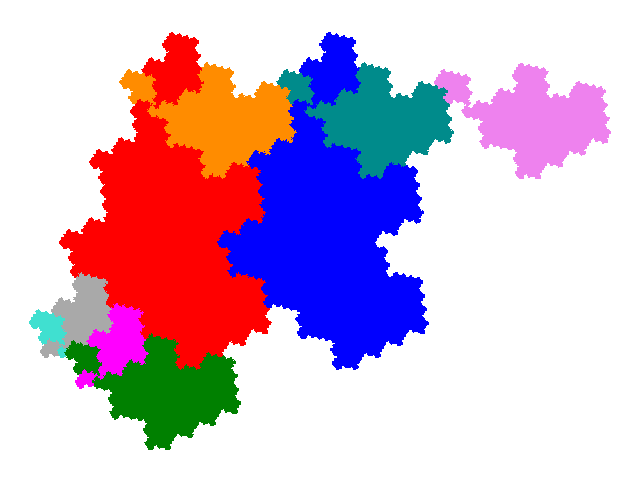The order 13 tiles are.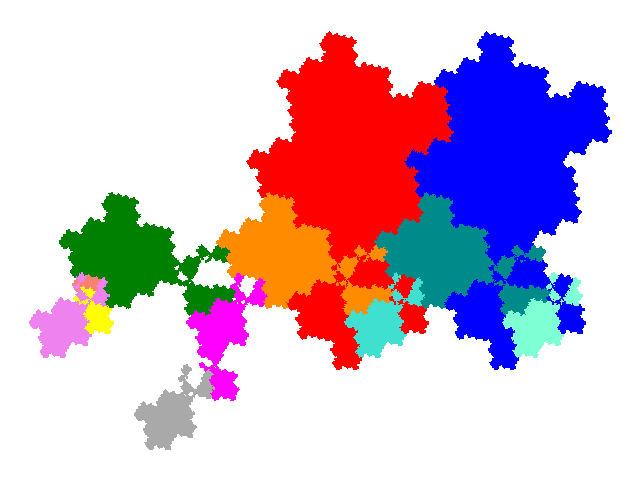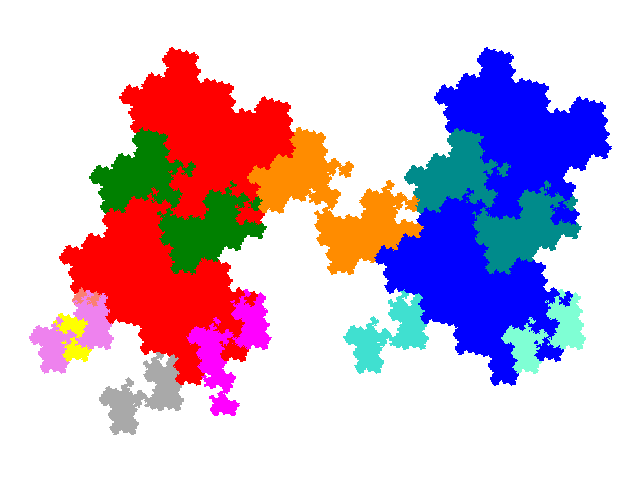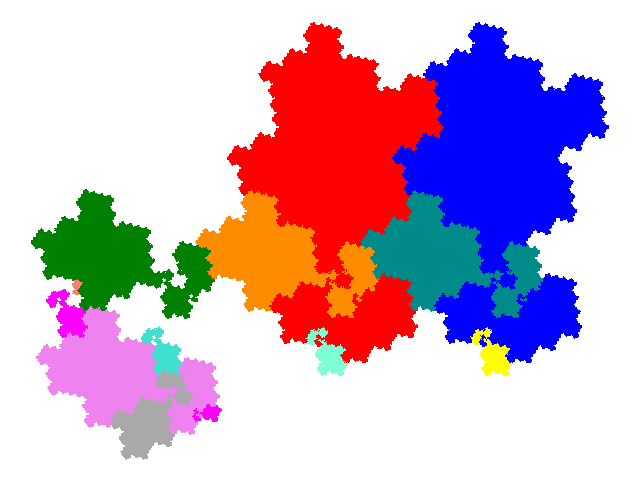All of these figures tile the plane. The order 9 tiles have 2 copies in the unit cell, and the order 13 tiles 3 copies in the unit cell. The tiling vectors are the same as for the order 5 tile.